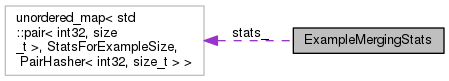ExampleMergingStats Class Reference

This class is responsible for storing, and displaying in log messages, statistics about how examples of different sizes (c.f. More...

`#include <nnet-example-utils.h>`

Collaboration diagram for ExampleMergingStats:[legend]

## Classes

struct  StatsForExampleSize

## Public Member Functions

void WroteExample (int32 example_size, size_t structure_hash, int32 minibatch_size)
Users call this function to inform this class that one minibatch has been written aggregating 'minibatch_size' separate examples of original size 'example_size' (e.g. More...

Users call this function to inform this class that after processing all the data, for examples of original size 'example_size', 'num_discarded' examples could not be put into a minibatch and were discarded. More...

void PrintStats () const
Calling this will cause a log message with information about the examples to be printed. More...

## Private Types

typedef unordered_map< std::pair< int32, size_t >, StatsForExampleSize, PairHasher< int32, size_t > > StatsType

## Private Member Functions

void PrintAggregateStats () const

void PrintSpecificStats () const

StatsType stats_

## Detailed Description

This class is responsible for storing, and displaying in log messages, statistics about how examples of different sizes (c.f.

GetNnetExampleSize()) were merged into minibatches, and how many examples were left over and discarded.

Definition at line 427 of file nnet-example-utils.h.

## ◆ StatsType

 typedef unordered_map, StatsForExampleSize, PairHasher > StatsType
private

Definition at line 464 of file nnet-example-utils.h.

## Member Function Documentation

Users call this function to inform this class that after processing all the data, for examples of original size 'example_size', 'num_discarded' examples could not be put into a minibatch and were discarded.

Definition at line 1065 of file nnet-example-utils.cc.

1067  {
1068  std::pair<int32, size_t> p(example_size, structure_hash);
1070 }

## ◆ PrintAggregateStats()

 void PrintAggregateStats ( ) const
private

Definition at line 1078 of file nnet-example-utils.cc.

1078  {
1079  // First print some aggregate stats.
1080  int64 num_distinct_egs_types = 0, // number of distinct types of input egs
1081  // (differing in size or structure).
1083  total_discarded_egs_size = 0, // total number of discarded egs each multiplied by size
1084  // of that eg
1085  total_non_discarded_egs = 0, // total over all minibatches written, of
1086  // minibatch-size, equals number of input egs
1087  // that were not discarded.
1088  total_non_discarded_egs_size = 0, // total over all minibatches of size-of-eg
1089  // * minibatch-size.
1090  num_minibatches = 0, // total number of minibatches
1091  num_distinct_minibatch_types = 0; // total number of combination of
1092  // (type-of-eg, number of distinct
1093  // minibatch-sizes for that eg-type)-
1094  // reflects the number of time we have
1095  // to compile.
1096
1097  StatsType::const_iterator eg_iter = stats_.begin(), eg_end = stats_.end();
1098
1099  for (; eg_iter != eg_end; ++eg_iter) {
1100  int32 eg_size = eg_iter->first.first;
1101  const StatsForExampleSize &stats = eg_iter->second;
1102  num_distinct_egs_types++;
1105
1106  unordered_map<int32, int32>::const_iterator
1107  mb_iter = stats.minibatch_to_num_written.begin(),
1108  mb_end = stats.minibatch_to_num_written.end();
1109  for (; mb_iter != mb_end; ++mb_iter) {
1110  int32 mb_size = mb_iter->first,
1111  num_written = mb_iter->second;
1112  num_distinct_minibatch_types++;
1113  num_minibatches += num_written;
1114  total_non_discarded_egs += num_written * mb_size;
1115  total_non_discarded_egs_size += num_written * mb_size * eg_size;
1116  }
1117  }
1118  // the averages are written as integers- we don't really need more precision
1119  // than that.
1121  total_input_egs_size =
1123
1124  float avg_input_egs_size = total_input_egs_size * 1.0 / total_input_egs;
1126  // note: by minibatch size we mean the number of egs per minibatch, it
1127  // does not take note of the size of the input egs.
1128  float avg_minibatch_size = total_non_discarded_egs * 1.0 / num_minibatches;
1129
1130  std::ostringstream os;
1131  os << std::setprecision(4);
1132  os << "Processed " << total_input_egs
1133  << " egs of avg. size " << avg_input_egs_size
1134  << " into " << num_minibatches << " minibatches, discarding "
1135  << percent_discarded << "% of egs. Avg minibatch size was "
1136  << avg_minibatch_size << ", #distinct types of egs/minibatches "
1137  << "was " << num_distinct_egs_types << "/"
1138  << num_distinct_minibatch_types;
1139  KALDI_LOG << os.str();
1140 }
kaldi::int32 int32
#define KALDI_LOG
Definition: kaldi-error.h:153

## ◆ PrintSpecificStats()

 void PrintSpecificStats ( ) const
private

Definition at line 1142 of file nnet-example-utils.cc.

1142  {
1143  KALDI_LOG << "Merged specific eg types as follows [format: <eg-size1>="
1145  ",<egs-size2>={...},... (note,egs-size == number of input "
1146  "frames including context).";
1147  std::ostringstream os;
1148
1149  // copy from unordered map to map to get sorting, for consistent output.
1150  typedef std::map<std::pair<int32, size_t>, StatsForExampleSize> SortedMapType;
1151
1152  SortedMapType stats;
1153  stats.insert(stats_.begin(), stats_.end());
1154  SortedMapType::const_iterator eg_iter = stats.begin(), eg_end = stats.end();
1155  for (; eg_iter != eg_end; ++eg_iter) {
1156  int32 eg_size = eg_iter->first.first;
1157  if (eg_iter != stats.begin())
1158  os << ",";
1159  os << eg_size << "={";
1160  const StatsForExampleSize &stats = eg_iter->second;
1161  unordered_map<int32, int32>::const_iterator
1162  mb_iter = stats.minibatch_to_num_written.begin(),
1163  mb_end = stats.minibatch_to_num_written.end();
1164  for (; mb_iter != mb_end; ++mb_iter) {
1165  int32 mb_size = mb_iter->first,
1166  num_written = mb_iter->second;
1167  if (mb_iter != stats.minibatch_to_num_written.begin())
1168  os << ",";
1169  os << mb_size << "->" << num_written;
1170  }
1171  os << ",d=" << stats.num_discarded << "}";
1172  }
1173  KALDI_LOG << os.str();
1174 }
kaldi::int32 int32
#define KALDI_LOG
Definition: kaldi-error.h:153

## ◆ PrintStats()

 void PrintStats ( ) const

Calling this will cause a log message with information about the examples to be printed.

Definition at line 1073 of file nnet-example-utils.cc.

## ◆ WroteExample()

 void WroteExample ( int32 example_size, size_t structure_hash, int32 minibatch_size )

Users call this function to inform this class that one minibatch has been written aggregating 'minibatch_size' separate examples of original size 'example_size' (e.g.

as determined by GetNnetExampleSize(), but the caller does that. The 'structure_hash' is provided so that this class can distinguish between egs that have the same size but different structure. In the extremely unlikely eventuality that there is a hash collision, it will cause misleading stats to be printed out.

Definition at line 1051 of file nnet-example-utils.cc.

1053  {
1054  std::pair<int32, size_t> p(example_size, structure_hash);
1055
1056
1057  unordered_map<int32, int32> &h = stats_[p].minibatch_to_num_written;
1058  unordered_map<int32, int32>::iterator iter = h.find(minibatch_size);
1059  if (iter == h.end())
1060  h[minibatch_size] = 1;
1061  else
1062  iter->second += 1;
1063 }

## ◆ stats_

 StatsType stats_
private

Definition at line 468 of file nnet-example-utils.h.

The documentation for this class was generated from the following files: